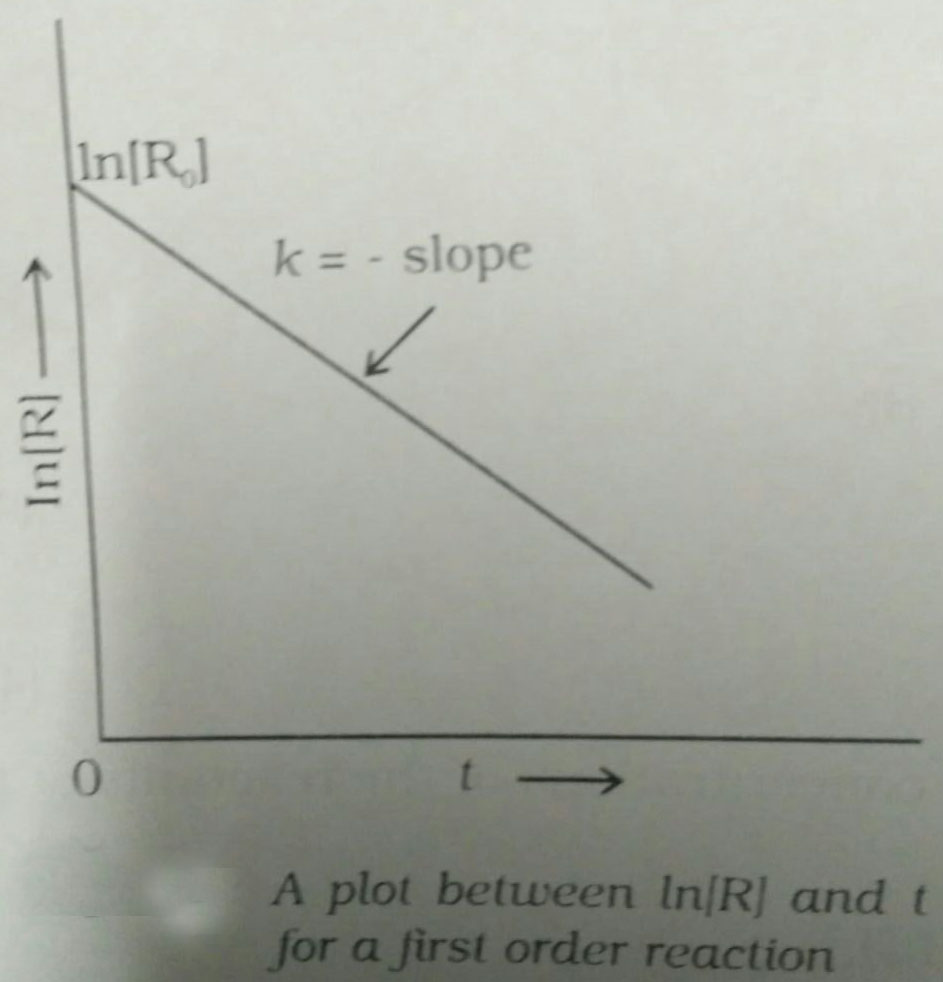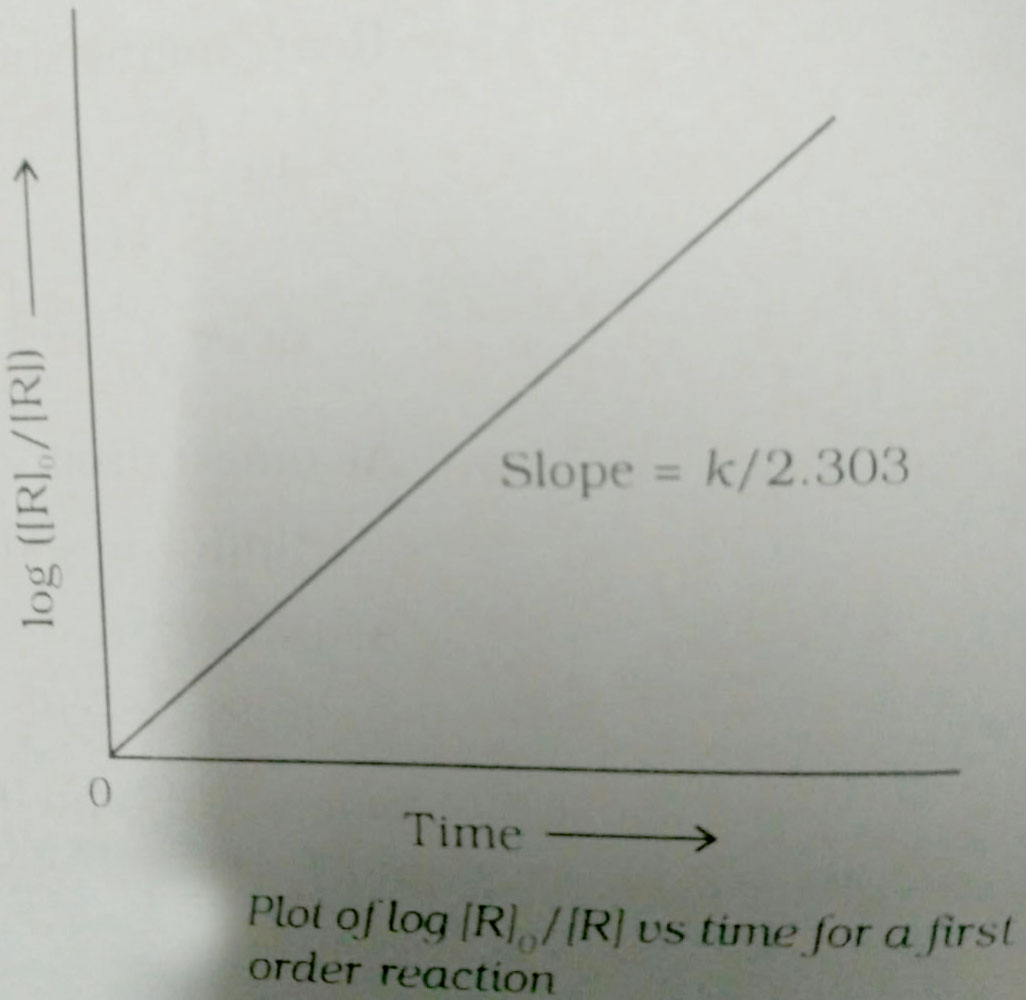#### units of rate constant of first and zero order reactions in terms of molarity M unit are respectively Option 1)Option 2)Option 3)Option 4)As we learnt in

Zero Order Reaction -

Zero order reaction means that the rate of the reaction is proportional to zeroth power of the concentration of reactants

- whereintime required for half completion of reactionFirst Order Reaction -

The rate of the reaction is proportional to the first power of the concentration of the reaction- wherein

Formula:

RP

a                 0

a-x             x[differentiate rate law]Unit offor zero order reactionunit of k isfor first order reactionunit ofOption 1)Correct option

Option 2)Incorrect option

Option 3)Incorrect option

Option 4)Incorrect option

#### Plabita